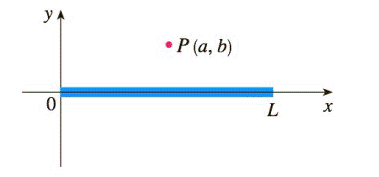Chapter 7.3, Problem 42E

Chapter
Section
Textbook Problem

# A charged rod of length L produces an electric field at point P(a, b) given by E ( P ) = ∫ − a L − a λ b 4 π ε 0 ( x 2 + b 2 ) 3 / 2   d x where λ is the charge density per unit length on the rod and ε 0 is the free space permittivity (see the figure). Evaluate the integral to determine an expression for the electric field E(P).To determine

To Find : The Value of the integral E(P)=aLaλb4πε0(x2+b2)32dx

Explanation

E(P)=λ4πbε0[Lab2+(La)2+ab2+a2]

Calculation: Substituting x=btanθ,dx=bsec2θdθ

Consider evaluating the limit without applying the limits first

E(P)=λb4πε0(b2tan2θ+b2)32bsec2θdθ=λ4πε0dθbsecθ=λ4πbε0cosθdθ=λ4πbε0sinθ

### Still sussing out bartleby?

Check out a sample textbook solution.

See a sample solution

#### The Solution to Your Study Problems

Bartleby provides explanations to thousands of textbook problems written by our experts, many with advanced degrees!

Get Started

#### Find more solutions based on key concepts